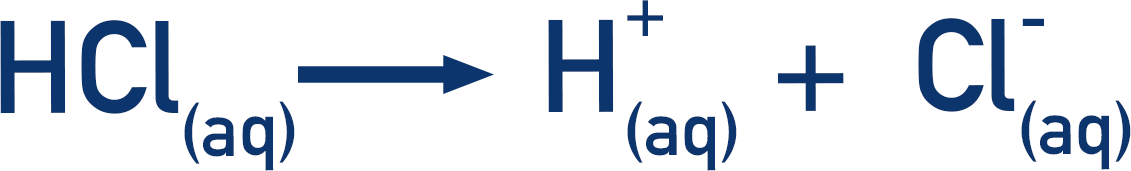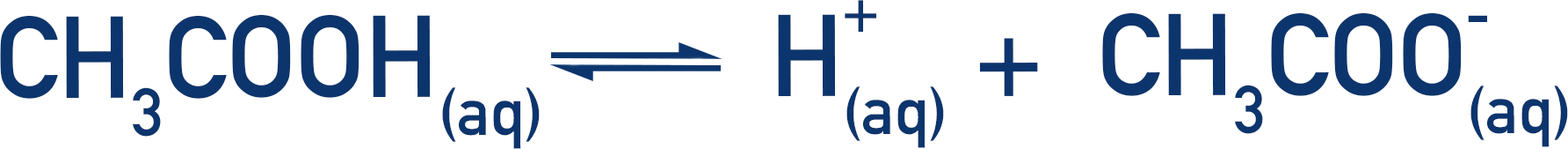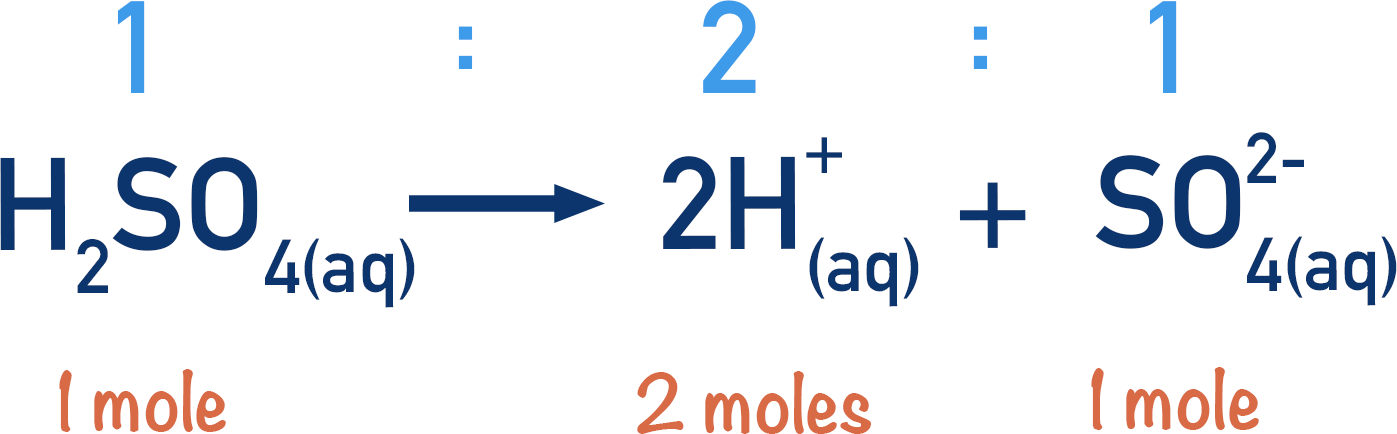Quick Notes Strong and Weak Acids

• Strong acids fully dissociate in solution – all molecules of the acid split, releasing the maximum number of protons, H+(aq), possible.
• Weak acids partially dissociate in solution – some acid molecules that have released a proton re-gain a proton to form a molecule of the acid again.
• Monoprotic acids release one H+(aq) ion for every one molecule of acid.
• Diprotic acids release two H+(aq) ions for every one molecule of acid.
• The concentration of a strong, monoprotic acid solution is the same as the concentration of H+(aq) ions present in the solution.

Full Notes Strong and Weak Acids

A strong acid fully dissociates in solution. This means all molecules of the acid in the solution release all the possible H+(aq) ions that they can. For example, hydrochloric acid.The number of moles of HCl(aq) that we start with is the same as the number of moles of H+(aq) that are released. The concentration of H+(aq) ions in solution is the same as the original concentration of acid.

## Weak Acids

Weak acids do not fully dissociate in solution, they partially dissociate. This means the concentration of H+(aq) ions in solution is not the same as the original concentration of acid.

Carboxylic acids are a common example of weak acids. Ethanoic acid is a weak acid that is very common in all A-level specifications.

When ethanoic acid (CH3COOH) is placed in water, some of the acid molecules will lose a proton (H+) to become negatively charged ethanoate ions (CH3COO-). Ethanoate ions are the conjugate base of ethanoic acid – see Acids and Bases.

Some ethanoate ions will then recombine with a proton, to reform the acid molecule. Meanwhile, more acid molecules will lose protons to become ethanoate ions. This means an equilibrium is formed. There will still always be acid molecules present in the solution, as well as protons and conjugate base ions.Note – this may seem very confusing at first, but it is important to understand that when we say weak acids do not fully dissociate what we really mean is there is a constant exchange of protons between acid molecules and their conjugate base ions. This constant exchange leads to an equilibrium being formed, where the overall concentrations of acid and conjugate base molecules do not change, but individual acids and bases are still exchanging protons. This is very important to understand in preparation for pH and buffer calculations.

## Monoprotic and Diprotic Acids

In the above example, the HCl(aq) releases one H+(aq) ion for every molecule of HCl. This is an example of a monoprotic acid. Mono means one, protic means proton. A monoprotic acid releases only one H+(aq) ion for every molecule of acid.

Some acids can release more than one H+(aq) ion per molecule. This is usually two protons and such acids are called diprotic acids. Sulfuric acid (H2SO4) is an example of a diprotic acid.One mole of a diprotic acid can release two moles of H+(aq) ions.

When attempting calculations with acids and bases – make sure you check the number of protons being released by an acid!